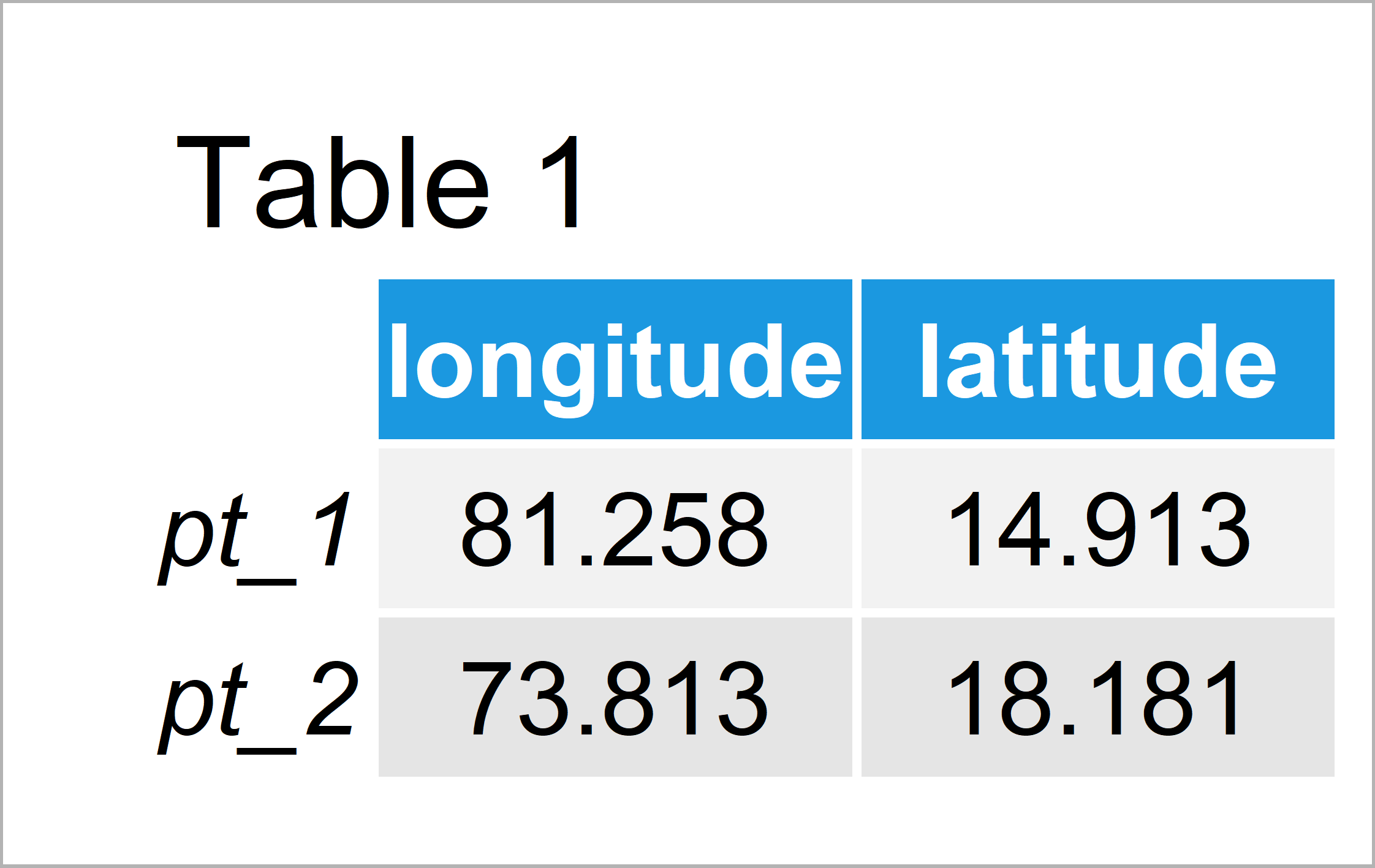# Haversine Great Circle Distance in R (Example)

This post explains how to calculate the Haversine distance in the R programming language.

Let’s dig in:

## Creation of Example Data

In the first place, we need to define some data that we can use in the following examples:

```my_points <- matrix(c(81.25798, 73.81277,    # Create longitude/latitude matrix
14.91254, 18.18145),
nrow = 2)
colnames(my_points) <- c("longitude", "latitude")
rownames(my_points) <- c("point_1", "point_2")
my_points                                    # Print longitude/latitude matrix```As you can see based on Table 1, our example data is a matrix consisting of two longitudinal and latitudinal data points.

## Example: Calculate Haversine Great Circle Distance Using distHaversine() Function of geosphere Package

In this example, I’ll show how to apply the distHaversine function of the geosphere package to calculate the Haversine distance of two geospatial points in R.

First, we need to install and load the geosphere package:

```install.packages("geosphere")                # Install & load geosphere
library("geosphere")```

Next, we can use the distHaversine function to get the distance between our two geographical points according to the Haversine formula:

```my_dist <- distHaversine(my_points)          # Calculate Haversine distance
my_dist                                      # Print Haversine distance
#  873680.6```

The Haversine distance between our points is 873680.6.

## Video, Further Resources & Summary

Have a look at the following video instruction of my YouTube channel. I’m explaining the R code of this article in the video.

In addition, you might have a look at some of the other tutorials on my website. Please find a selection of tutorials about distance measures here:

Summary: You have learned in this tutorial how to compute the Haversine great circle distance in R programming. Don’t hesitate to let me know in the comments below, if you have further questions. In addition, don’t forget to subscribe to my email newsletter in order to get updates on the newest tutorials.

Subscribe to the Statistics Globe Newsletter

•Stefan Dillinger
April 14, 2023 7:29 pm

Hi Joachim,

i have a list of 472 points with longitude and latitude and I want to calculate the distance of those points from home. I tried to use the knowledge above to do that, but the distances seem off. I made an excel list with the coordinates
longitude1 latitude1 longitude2 latitude2

and expected to get the distance for each row… However, that does not seem to be the case.

Can you help me to apply the distHaversine on more than one pair of coordinates?

Thanks,
Stefan

•April 18, 2023 1:09 pm

Hello Stefan,

The reason that you have trouble is due to the fact that this function is only used for calculating the distance between two data points. You can used the defined function below instead. I assigned some arbitrary numbers for the point coordinates and home coordinates.

```distHaversine <- function(lon1, lat1, lon2, lat2) {
R <- 6371  # Earth's radius in km
dLat <- (lat2 - lat1) * pi / 180
dLon <- (lon2 - lon1) * pi / 180
a <- sin(dLat/2) * sin(dLat/2) +
cos(lat1 * pi / 180) * cos(lat2 * pi / 180) *
sin(dLon/2) * sin(dLon/2)
c <- 2 * atan2(sqrt(a), sqrt(1-a))
d <- R * c
return(d)
}

# Set home coordinates
home_lon <- -122.4194
home_lat <- 37.7749

# Create a data frame of coordinates
coordinates <- data.frame(
lon = c(-122.4194, -122.4088, -122.4156, -122.4102),
lat = c(37.7749, 37.7837, 37.7937, 37.7943)
)

# Calculate distances from home
distances <- apply(coordinates, 1, function(row) {
distHaversine(home_lon, home_lat, row["lon"], row["lat"])
})

# Print distances
print(distances)```

Regards,
Cansu